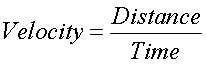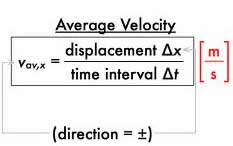# Difference between Velocity and Average Velocity

##### Key Difference: Velocity refers to the rate of change of distance with respect to time. It is a vector quantity, which means that it has both - a direction and a magnitude. Average velocity refers to the average total displacement over the time of travel. It is calculated by determining the average of inital and final velocities. The change in position can also be referred to as the displacement.Velocity denotes the rate of change of distance with respect to time. It is a vector quantity, which means that it has both - a direction and a magnitude. It can also be referred to as a speed with a direction. Velocity keeps the track of direction, and thus direction plays a vital role in the calculation of the velocity. One needs to specify the direction in order to provide the complete information regarding the velocity of an object. The units of velocity are m/sec, cm/sec, ft/sec, km/h, miles/hr, etc.Average velocity refers to the average change in position over the time of travel. It particularly defines the velocity of an object when it moves at the same speed and direction over time. If an object keeps on changing direction than initial direction of movement of the object is considered as the reference point. The change in position can also be referred to as the displacement. It is simply calculated by dividing the total displacement by the total time of travel. The net displacement is calculated by finding out the projections of the distaces in the direction of initial distance. Average velocity does not provide any information about the activity that takes place between the starting and ending point. It is also a vector quantity. If final and initial velocities are defined then the average velocity is calculated as (initial velocity + final velocity)/2.

Comparison between Velocity and Average Velocity:

 Velocity Average Velocity Definition Velocity refers to the rate of change of distance with respect to time. It is a vector quantity, which means that it has both - a direction and a magnitude Average velocity refers to the average total displacement over the time of travel. Formula Velocity = displacement/time Average Velocity = Total displacement/time interval Units m/sec, cm/sec, ft/sec, km/h, miles/hr, etc. m/sec, cm/sec, ft/sec, km/h, miles/hr, etc. Example If a car comes back to its initial position, then the displacement is zero. Therefore, this motion results in zero velocity. If a particle is moving along the x axis and is located at 10 metres at 2 seconds and at 12 metres at 4 seconds, then the average velocity in that particular time interval would be = (12-10)/(4-2 )= 5 m/s Purpose Velocity tells you that how fast and in what direction, an object is moving. When there are displacements corresponding to the different time taken, then we need to determine the average velocity.

Image Courtesy: waiferx.blogspot.com, astronomyonline.org